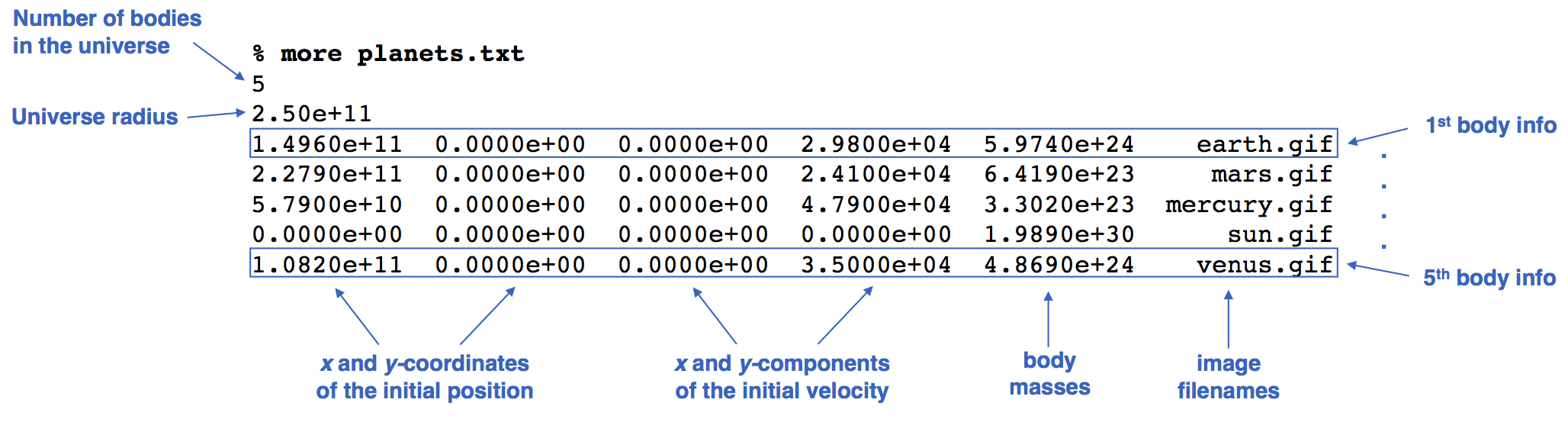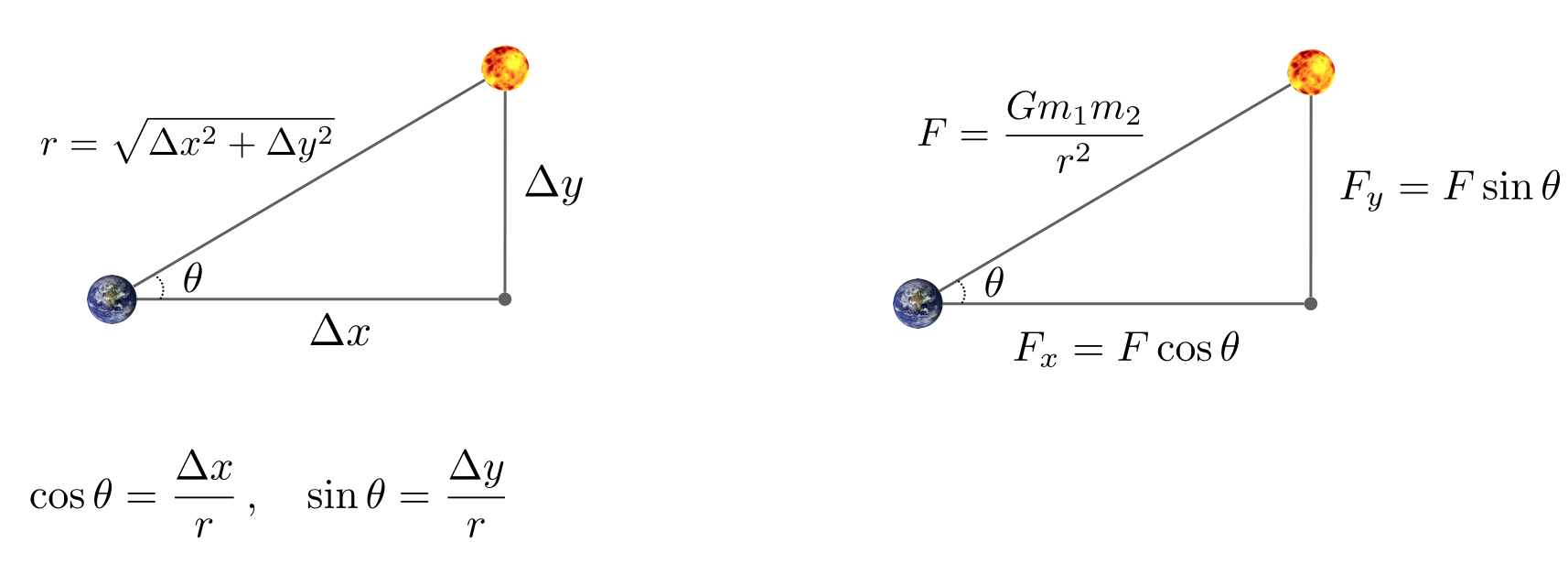## N-Body Simulation

Start: 10/8/2019
Due: 10/18/2019, by the beginning of class
Collaboration: individual

This assignment was developed by Robert Sedgewick and Kevin Wayne, Princeton University. Original assignment was in Java using their stdlib library.

### Goal

Write a Kotlin program using the Processing and Minim libraries to simulate the motion of n particles in the plane, mutually affected by gravitational forces, and animate the results. Such methods are widely used in cosmology, semiconductors, and fluid dynamics to study complex physical systems. Scientists also apply the same techniques to other pairwise interactions including Coulombic, Biot–Savart, and van der Waals.

### Context

In 1687, Isaac Newton formulated the principles governing the motion of two particles under the influence of their mutual gravitational attraction in his famous Principia. However, Newton was unable to solve the problem for three particles. Indeed, in general, solutions to systems of three or more particles must be approximated via numerical simulations.

### Program specification

Write a Kotlin program Nbody.kt that

• Takes one command-line argument—the name of the input file describing the universe to be simulated.
• Reads in the details of the universe to be simulated from the input file using java.util.Scanner, using several parallel arrays to store the data.
• Simulates the universe, using the leapfrog scheme described below.
• Animates the results using the Processing library.

### Input format

The program’s input is a text file that contains the information for a particular universe (in SI units, using the Cartesian Coordinate system).

• The first value is an integer n which represents the number of particles. The second value is a real number radius which represents the radius of the universe; it is used to determine the necessary amount of scaling (and transformation) to convert between the Cartesian coordinate system and the drawing window’s coordinate system. (The drawing window must display particles with their Cartesian x- and y-coordinates between −radius and +radius.)
• Next, there are n lines (one for each particle), with each line containing 6 values. The first two values are the Cartesian x- and y-coordinates of the initial position; the next pair of values are the Cartesian x- and y-components of the initial velocity; the fifth value is the mass; the last value is a String that is the name of an image file used to display the particle.
• The remainder of the file (optionally) contains a description of the universe, which your program must ignore.

As an example, planets.txt contains real data from part of our Solar System.### Getting started

Before you begin coding, do the following.

• Get familiar with using gradle. Learn the rudiments of gradle and read the gradle howto guide.

• Get familiar with the relevant part of the Processing core library and the Minim sound library.

• Download this zipped gradle project nbody.zip and unzip it into your ~/mcs178 directory. To test your program, you will need planets.txt and the accompanying sound file and image files. The root directory of the zip file nbody.zip contains a directory named worlds which in turn contains planets.txt and additional test universes. The image and sound files are in the directory src/resources. The root directory also contains a readme.txt template for you to fill out and submit along with your Kotlin code. We also provide the skeleton code in the file src/main/kotlin/Nbody.kt. All you have to do is to fill out the code for this one file.

### Compiling and executing your program

I’m assuming you have unzipped the provided file nbody.zip into your ~/mcs178 directory, and your shell’s current directory is ~/mcs178/nbody. See zip how-to.

To compile your program using gradle, type the following in your terminal application (linux terminal or OS X Terminal):

$gradle compileKotlin To execute your program using gradle, and use worlds/planets.txt as input file, type: $ gradle run --args=worlds/planets.txt

### Simulating the universe: the physics

We review the equations governing the motion of the particles, according to Newton's laws of motion and gravitation. Don't worry if your physics is a bit rusty; all of the necessary formulas are included below. We'll assume for now that the position (px, py) and velocity (vx, vy) of each particle is known. In order to model the dynamics of the system, we must know the net force exerted on each particle.

• Pairwise force. Newton's law of universal gravitation asserts that the strength of the gravitational force between two particles is given by the product of their masses divided by the square of the distance between them, scaled by the gravitational constant G (6.67 × 10 −11 N·m 2·kg −2). The pull of one particle towards another acts on the line between them. Since we are using Cartesian coordinates to represent the position of a particle, it is convenient to break up the force into its x- and y-components (Fx, Fy) as illustrated here.• Net force. The principle of superposition says that the net force acting on a particle in the x- or y-direction is the sum of the pairwise forces acting on the particle in that direction.
• Acceleration. Newton's second law of motion postulates that the accelerations in the x- and y-directions are given by: ax = Fx / m, ay = Fy / m.

### Simulating the universe: the numerics

We use the leapfrog finite difference approximation scheme to numerically integrate the above equations: this is the basis for most astrophysical simulations of gravitational systems. In the leapfrog scheme, we discretize time, and update the time variable t in increments of the time quantum Δt (measured in seconds). We maintain the position (px, py) and velocity (vx, vy) of each particle at each time step. The steps below illustrate one possible way to evolve the positions and velocities of the particles.

• Step A (calculate the forces). For each particle, calculate the net force (Fx, Fy) at the current time t acting on that particle using Newton's law of gravitation and the principle of superposition. Note that force is a vector (i.e., it has direction). In particular, Δx and Δy are signed (positive or negative). In the diagram above, when you compute the force the sun exerts on the earth, the sun is pulling the earth up (Δy positive) and to the right (Δx positive).
• Step B (update the velocities and positions). For each particle:
1. Calculate its acceleration (ax, ay) at time t using the net force computed in Step A and Newton's second law of motion: ax = Fx / m, ay = Fy / m.
2. Calculate its new velocity (vx, vy) at the next time step by using the acceleration computed in (i) and the velocity from the old time step: Assuming the acceleration remains constant in this interval, the new velocity is (vx + Δt ax, vy + Δt ay).
3. Calculate its new position (px, py) at time t + Δt by using the velocity computed in (ii) and its old position at time t: Assuming the velocity remains constant in this interval, the new position is (px + Δt vx, py + Δt vy).
• Step C (draw the universe).  Draw each particle, using the position computed in Step B.

Do not interleave steps A and B(iii); otherwise, you will be computing the forces at time t using the positions of some of the particles at time t and others at time t + Δt. The simulation is more accurate when Δt is very small, but this comes at the price of more computation.

### Finishing touches

The first finishing touch is to play the theme to 2001: A Space Odyssey using the Minim library and the audio file src/resources/2001.wav. The second is to find a free starfield image and learn how to use it in your own project. The image should be interesting and bigger than the given starfield.jpg. In fact, its size should be as close to your computer display size as possible.

### Challenge for the bored

There are limitless opportunities for additional excitement and discovery here. Create an alternate universe (using the same input format). Try adding other features, such as supporting elastic or inelastic collisions. Or, make the simulation three-dimensional by doing calculations for x-, y-, and z-coordinates, then using the z-coordinate to vary the sizes of the planets. Add a rocket ship that launches from one planet and has to land on another. Allow the rocket ship to exert force with the consumption of fuel.

Zip up your Nbody.kt and the completed readme.txt files and submit via Moodle. See zip how-to for how to zip up files.